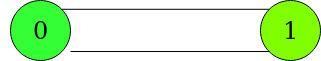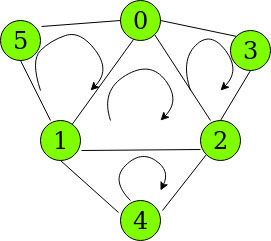# Count of all cycles without any inner cycle in a given Graph

Given an undirected graph consisting of N vertices numbered [0, N-1] and E edges, the task is to count the number of cycles such that any subset of vertices of a cycle does not form another cycle.

Examples:

Input: N = 2, E = 2, edges = [{0, 1}, {1, 0}]
Output: 1
Explanation:
Only one cycle exists between the two vertices.Input: N = 6, E = 9, edges = [{0, 1}, {1, 2}, {0, 2}, {3, 0}, {3, 2}, {4, 1}, {4, 2}, {5, 1}, {5, 0}]
Output: 4
Explanation:
The possible cycles are shown in the diagram below:Cycles such as 5 -> 0 -> 2 -> 1 -> 5 are not considered as it comprises of inner cycles {5 -> 0 -> 1} and {0 -> 1 -> 2}

Approach:
Since V vertices require V edges to form 1 cycle, these the number of required cycles can be expressed using the formula:

```(Edges - Vertices) + 1
```

Illustration:

N = 6, E = 9, edges = [{0, 1}, {1, 2}, {0, 2}, {3, 0}, {3, 2}, {4, 1}, {4, 2}, {5, 1}, {5, 0}]
Number of Cycles = 9 – 6 + 1 = 4
The 4 cycles in the graph are:
{5, 0, 1}, {0, 1, 2}, {3, 0, 2} and {1, 2, 4}

This formula also covers the case when a single vertex may have a self-loop.

Below is the implementation of the above approach:

## C++

 `// C++ implementation for the ` `// above approach. ` ` `  `#include ` `using` `namespace` `std; ` ` `  `// Function to return the ` `// count of required cycles ` `int` `numberOfCycles(``int` `N, ``int` `E, ` `                   ``int` `edges[]) ` `{ ` `    ``vector<``int``> graph[N]; ` `    ``for` `(``int` `i = 0; i < E; i++) { ` `        ``graph[edges[i]] ` `            ``.push_back(edges[i]); ` `        ``graph[edges[i]] ` `            ``.push_back(edges[i]); ` `    ``} ` ` `  `    ``// Return the number of cycles ` `    ``return` `(E - N) + 1; ` `} ` ` `  `// Driver Code ` `int` `main() ` `{ ` `    ``int` `N = 6; ` `    ``int` `E = 9; ` `    ``int` `edges[] = { { 0, 1 }, ` `                       ``{ 1, 2 }, ` `                       ``{ 2, 0 }, ` `                       ``{ 5, 1 }, ` `                       ``{ 5, 0 }, ` `                       ``{ 3, 0 }, ` `                       ``{ 3, 2 }, ` `                       ``{ 4, 2 }, ` `                       ``{ 4, 1 } }; ` `    ``int` `k = numberOfCycles(N, E, ` `                           ``edges); ` ` `  `    ``cout << k << endl; ` `    ``return` `0; ` `} `

Output:

```4
```

Time Complexity: O(E)
Auxiliary Space: O(N)

Don’t stop now and take your learning to the next level. Learn all the important concepts of Data Structures and Algorithms with the help of the most trusted course: DSA Self Paced. Become industry ready at a student-friendly price.

My Personal Notes arrow_drop_upCheck out this Author's contributed articles.

If you like GeeksforGeeks and would like to contribute, you can also write an article using contribute.geeksforgeeks.org or mail your article to contribute@geeksforgeeks.org. See your article appearing on the GeeksforGeeks main page and help other Geeks.

Please Improve this article if you find anything incorrect by clicking on the "Improve Article" button below.